Home > AC > Chapter 5 > Lesson 5.2.2 > Problem5-80

5-80.
1. The graph below contains the lines for y = x + 2 and y = 2x − 1. Homework Help ✎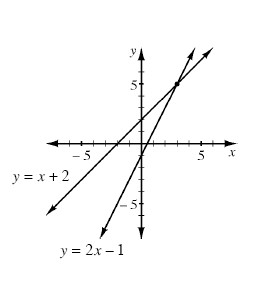1. Using the graph, what is the solution to this system?

2. Solve the system algebraically to confirm your answer to part (a).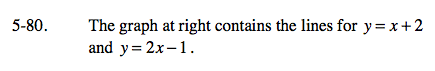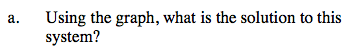What is the point at which the two lines intersect?

(3, 5)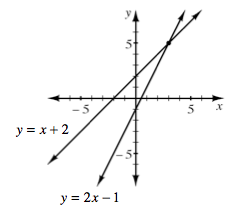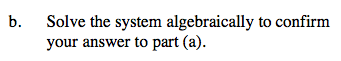Use the Equal Values method by setting the equations equal to each other.

Did you get the same solutions for x and y as in part (a)?